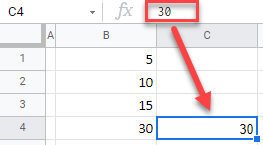# Copy Number Not Formula – Excel & Google Sheets

This tutorial demonstrates how to copy a number without its formula in Excel and Google Sheets.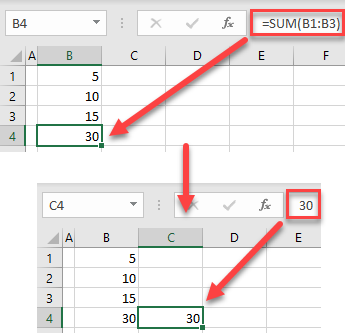## Copy-Paste Values

In Excel, if you copy a cell containing a formula to another location, using simply copy-paste, it copies the formula, not the value. Say you have the following formula in cell B4.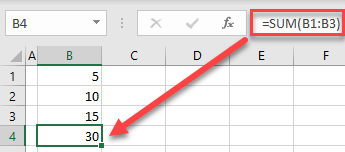The result of the SUM Function in cell B4 is 30. If you want to copy this number to cell C4 and not the formula, follow these steps:

1. Right-click the cell you want to copy (B4), and click Copy (or use the keyboard shortcut CTRL + C).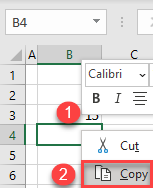1. Right-click the cell where you want to paste the number (C4), and click the Paste values icon (or use the keyboard shortcut).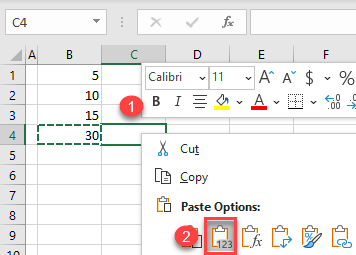As a result, only the number, and not the formula, is pasted.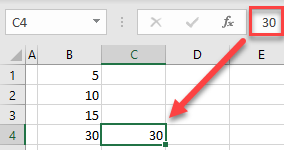Note: If you use the simple paste (CTRL + V), the formula from B4 is copied with updated relative cell references (C1:C3), and the result is 0.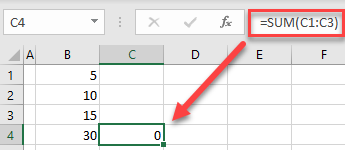## Copy-Paste Values in Google Sheets

In a similar way, you can copy just the number in Google Sheets.

1. Right-click the cell you want to copy (B4), and click Copy (or use the keyboard shortcut CTRL + C).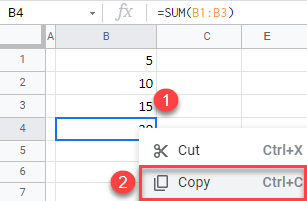1. Right-click the cell where you want to paste a number (C4), click Paste special, and choose Values only (or use the keyboard shortcut CTRL + SHIFT + V).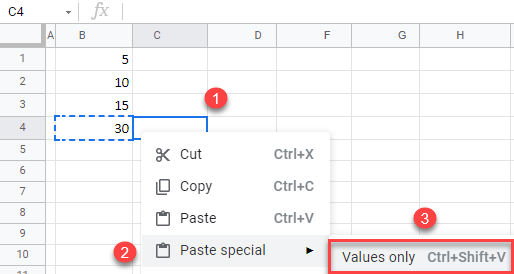As a result, only the result of the formula (30) is copied from B4 to C4.